## 12-2 MFCC

1. 預強調（Pre-emphasis）：將語音訊號 s(n) 通過一個高通濾波器：
H(z)=1-a*z-1
其中 a 介於 0.9 和 1.0 之間。若以時域的運算式來表示，預強調後的訊號 s2(n) 為
s2(n) = s(n) - a*s(n-1)
這個目的就是為了消除發聲過程中聲帶和嘴唇的效應，來補償語音信號受到發音系統所壓抑的高頻部分。（另一種說法則是要突顯在高頻的共振峰。）下面這個範例可以示範預強調所產生的效果：

Example 1: preEmphasis01.mwaveFile='whatFood.wav'; au=myAudioRead(waveFile); y=au.signal; fs=au.fs a=0.95; y2 = filter([1, -a], 1, y); time=(1:length(y))/fs; au2=au; au2.signal=y2; myAudioWrite(au2, 'whatFood_preEmphasis.wav'); subplot(2,1,1); plot(time, y); title('Original wave: s(n)'); subplot(2,1,2); plot(time, y2); title(sprintf('After pre-emphasis: s_2(n)=s(n)-a*s(n-1), a=%f', a)); subplot(2,1,1); set(gca, 'unit', 'pixel'); axisPos=get(gca, 'position'); uicontrol('string', 'Play', 'position', [axisPos(1:2), 60, 20], 'callback', 'sound(y, fs)'); subplot(2,1,2); set(gca, 'unit', 'pixel'); axisPos=get(gca, 'position'); uicontrol('string', 'Play', 'position', [axisPos(1:2), 60, 20], 'callback', 'sound(y2, fs)'); fs = 22050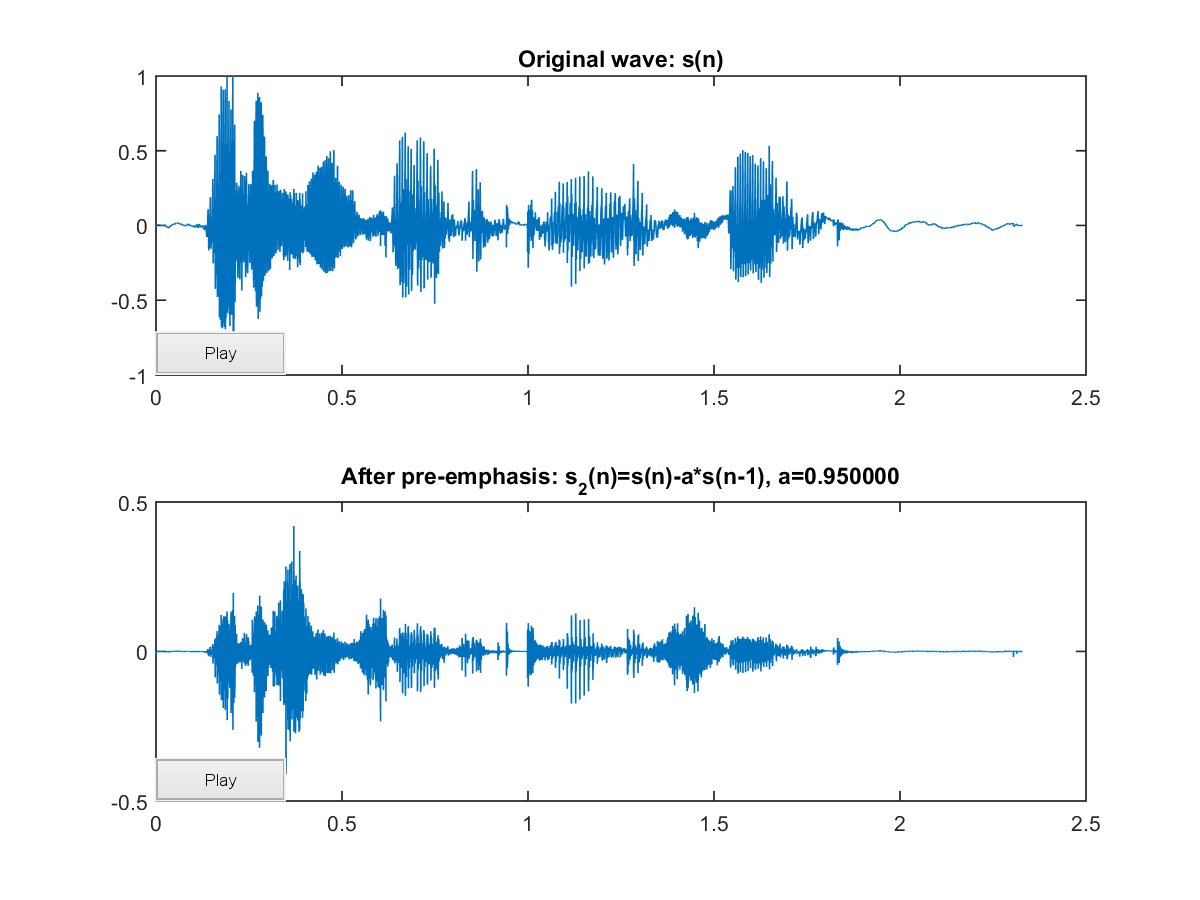很明顯地，經過了預強調之後，聲音變的比較尖銳清脆，但是音量也變小了。
2. 音框化（Frame blocking）：先將 N 個取樣點集合成一個觀測單位，稱為音框（Frame），通常 N 的值是 256 或 512，涵蓋的時間約為 20~30 ms 左右。為了避免相鄰兩音框的變化過大，所以我們會讓兩相鄰因框之間有一段重疊區域，此重疊區域包含了 M 個取樣點，通常 M 的值約是 N 的一半或 1/3。通常語音辨識所用的音訊的取樣頻率為 8 KHz或 16 KHz，以 8 KHz 來說，若音框長度為 256 個取樣點，則對應的時間長度是 256/8000*1000 = 32 ms。
3. 漢明窗（Hamming window）：將每一個音框乘上漢明窗，以增加音框左端和右端的連續性（請見下一個步驟的說明）。假設音框化的訊號為 S(n), n = 0,…N-1。那麼乘上漢明窗後為 S'(n) = S(n)*W(n)，此 W(n) 形式如下：
W(n, a) = (1 - a) - a cos(2pn/(N-1))，0≦n≦N-1
不同的 a 值會產生不同的漢明窗，圖形如下：

Example 2: hammingWindow01.m% Plot of generalized Hamming windows N=100; n=(0:N-1)'; alpha=linspace(0,0.5,11)'; h=[]; for i=1:length(alpha), h = [h, (1-alpha(i))-alpha(i)*cos(2*pi*n/(N-1))]; end plot(h); title('Generalized Hamming Window: (1-\alpha)-\alpha*cos(2\pin/(N-1)), 0\leqn\leqN-1'); legendStr={}; for i=1:length(alpha), legendStr={legendStr{:}, ['\alpha=', num2str(alpha(i))]}; end legend(legendStr);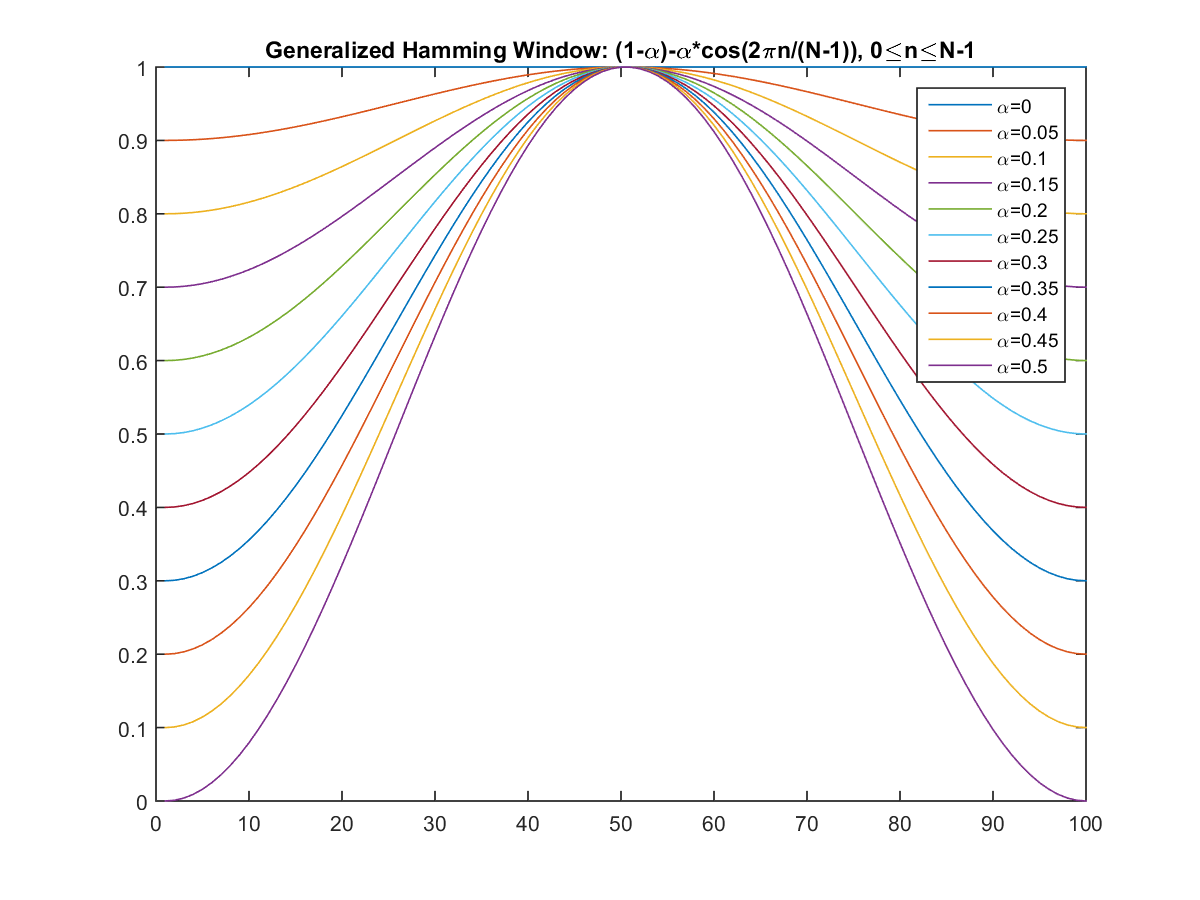一般我們都取 a = 0.46。（MATLAB 也提供了 hamming 指令，以產生漢明窗曲線。）
4. 快速傅利葉轉換（Fast Fourier Transform, or FFT）：由於訊號在時域（Time domain）上的變化通常很難看出訊號的特性，所以通常將它轉換成頻域（Frequency domain）上的能量分佈來觀察，不同的能量分佈，就能代表不同語音的特性。所以在乘上漢明窗後，每個音框還必需再經過 FFT 以得到在頻譜上的能量分佈。

乘上漢明窗的主要目的，是要加強音框左端和右端的連續性，這是因為在進行 FFT 時，都是假設一個音框內的訊號是代表一個週期性訊號，如果這個週期性不存在，FFT 會為了要符合左右端不連續的變化，而產生一些不存在原訊號的能量分佈，造成分析上的誤差。當然，如果我們在取音框時，能夠使音框中的訊號就已經包含基本週期的整數倍，這時候的音框左右端就會是連續的，那就可以不需要乘上漢明窗了。但是在實作上，由於基本週期的計算會需要額外的時間，而且也容易算錯，因此我們都用漢明窗來達到類似的效果。下面這個範例，顯示乘上漢明窗的效果：

Example 3: windowing01.mfs=8000; t=(1:512)'/fs; f=306.396; original=sin(2*pi*f*t)+0.2*randn(length(t),1); windowed=original.*hamming(length(t)); [mag1, phase1, freq1]=fftOneSide(original, fs); [mag2, phase2, freq2]=fftOneSide(windowed, fs); subplot(3,2,1); plot(t, original); grid on; axis([-inf inf -1.5 1.5]); title('Original signal'); subplot(3,2,2); plot(t, windowed); grid on; axis([-inf inf -1.5 1.5]); title('Windowed signal'); subplot(3,2,3); plot(freq1, mag1); grid on; title('Energy spectrum (linear scale)'); subplot(3,2,4); plot(freq2, mag2); grid on; title('Energy spectrum (linear scale)'); subplot(3,2,5); plot(freq1, 20*log10(mag1)); grid on; axis([-inf inf -20 60]); title('Energy spectrum (db)'); subplot(3,2,6); plot(freq2, 20*log10(mag2)); grid on; axis([-inf inf -20 60]); title('Energy spectrum (db)');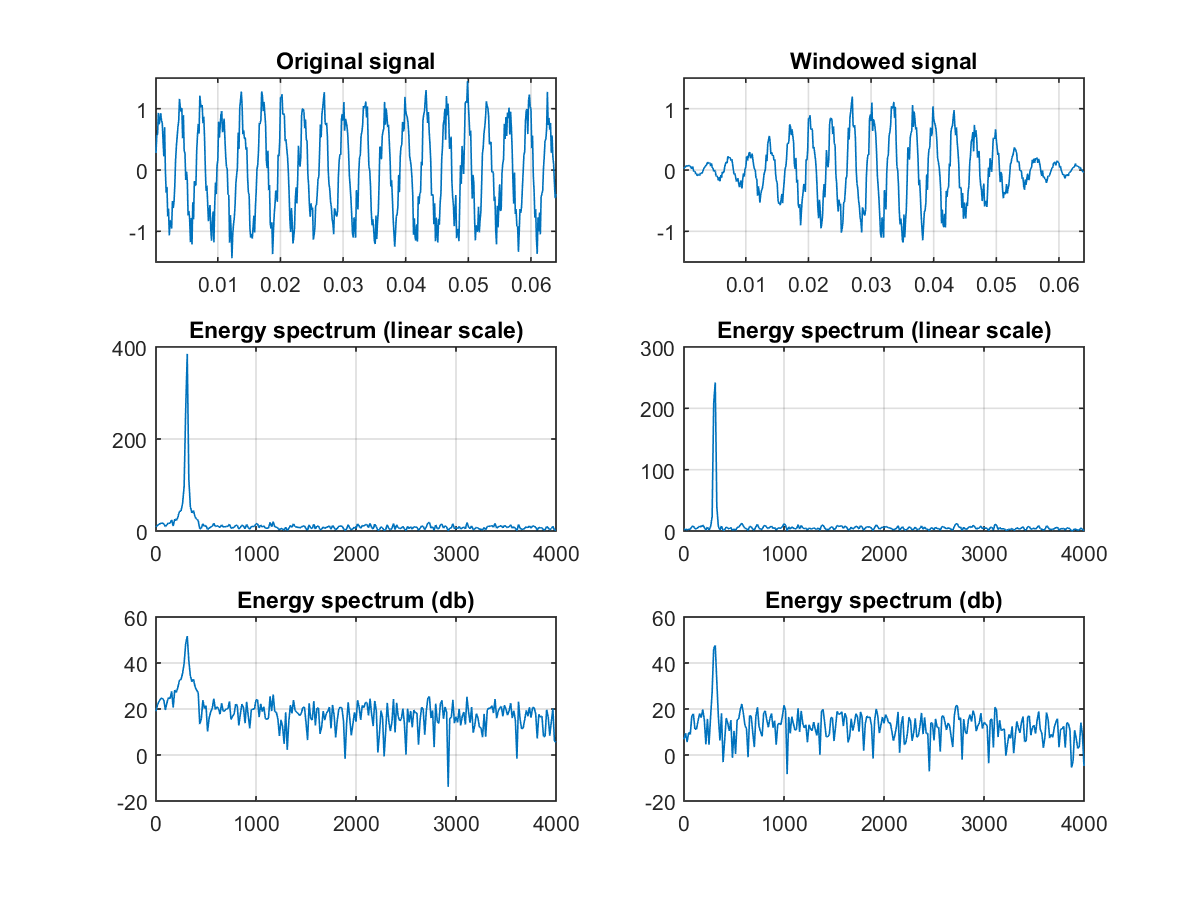在上述範例中，音框中的訊號是一段弦波加上雜訊，若不乘上漢明窗，音框的左端和右端並不連續，因此在頻譜上，代表弦波的高峰比較不明顯。若乘上漢明窗後，雜訊在能量頻譜上面的強度就會比較弱，代表弦波的高峰也相對比較突出。如果我們使用實際的聲音訊號來進行測試，漢明窗的效果就會更明顯：

Example 4: windowing02.mwaveFile='littleStar.wav'; au=myAudioRead(waveFile); y=au.signal; fs=au.fs; nbits=au.nbits; n=512; t=(1:n)'/fs; startIndex=30418; endIndex=startIndex+n-1; original=y(startIndex:endIndex); windowed=original.*hamming(n); [mag1, phase1, freq1]=fftOneSide(original, fs); [mag2, phase2, freq2]=fftOneSide(windowed, fs); subplot(3,2,1); plot(original); grid on; axis([-inf inf -1 1]); title('Original signal'); subplot(3,2,2); plot(windowed); grid on; axis([-inf inf -1 1]); title('Windowed signal'); subplot(3,2,3); plot(freq1, mag1); grid on; title('Energy spectrum (linear scale)'); subplot(3,2,4); plot(freq2, mag2); grid on; title('Energy spectrum (linear scale)'); subplot(3,2,5); plot(freq1, 20*log(mag1)); grid on; axis([-inf inf -80 120]); title('Energy spectrum (db)'); subplot(3,2,6); plot(freq2, 20*log(mag2)); grid on; axis([-inf inf -80 120]); title('Energy spectrum (db)');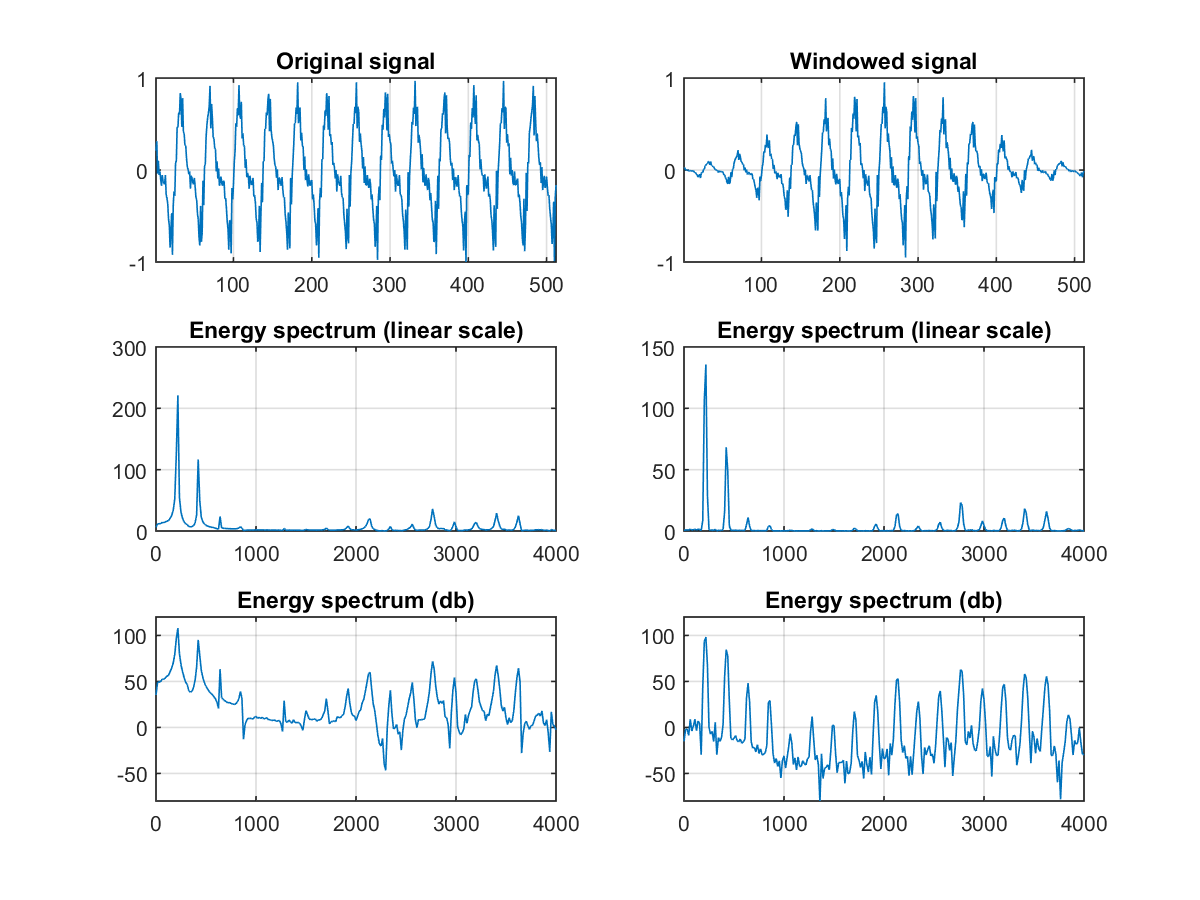在上述範例中，我們是用一段歌聲來分析，在乘上漢明窗之後，頻譜的諧波結構就變得非常明顯。
5. 三角帶通濾波器（Triangular Bandpass Filters）：將能量頻譜能量乘以一組 20 個三角帶通濾波器，求得每一個濾波器輸出的對數能量（Log Energy）。必須注意的是：這 20 個三角帶通濾波器在「梅爾頻率」（Mel Frequency）上是平均分佈的，而梅爾頻率和一般頻率 f 的關係式如下：
mel(f)=2595*log10(1+f/700)
或是
mel(f)=1125*ln(1+f/700)
梅爾頻率代表一般人耳對於頻率的感受度，由此也可以看出人耳對於頻率 f 的感受是呈對數變化的：
• 在低頻部分，人耳感受是比較敏銳
• 在高頻部分，人耳的感受就會越來越粗糙
以下這個範例，畫出一般頻率對於梅爾頻率的特性曲線：

Example 5: showMelFreq01.mlinFreq=0:8000; melFreq=lin2melFreq(linFreq); plot(linFreq, melFreq); xlabel('Frequency'); ylabel('Mel-frequency'); title('Frequency to mel-frequency curve'); axis equal tight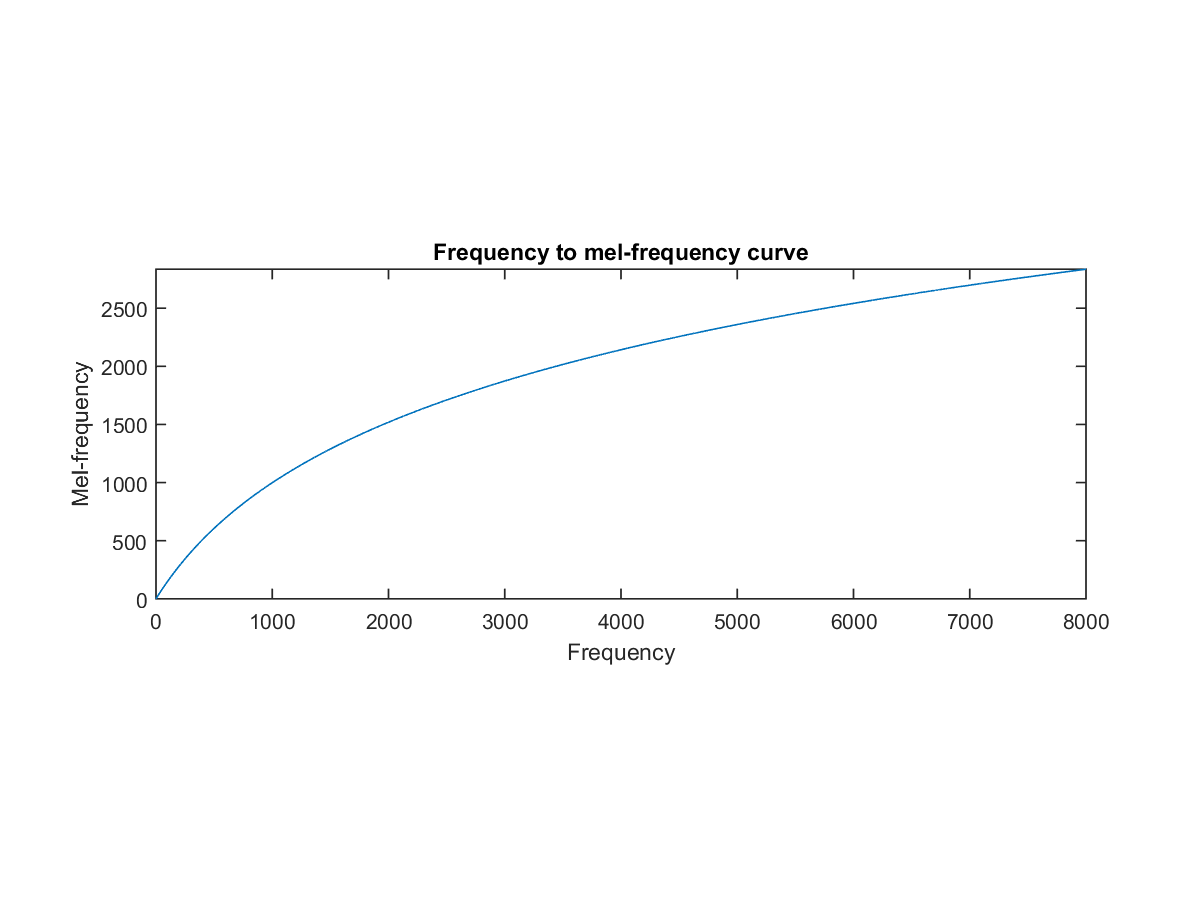在實作上，三角帶通濾波器的實現有兩種方式，請見下列範例：

Example 6: showTriFilterBank01.mfs=16000; filterNum=20; plotOpt=1; getTriFilterBankPrm(fs, filterNum, plotOpt);

三角帶通濾波器有兩個主要目的：
• 對頻譜進行平滑化，並消除諧波的作用，突顯原先語音的共振峰。（因此一段語音的音調或音高，是不會呈現在 MFCC 參數內，換句話說，以 MFCC 為特徵的語音辨識系統，並不會受到輸入語音的音調不同而有所影響。）
• 降低資料量。
6. 離散餘弦轉換（Discrete cosine transform, or DCT）：將上述的 20 個對數能量 Ek帶入離散餘弦轉換，求出 L 階的 Mel- scale Cepstrum 參數，這裡 L 通常取 12。離散餘弦轉換公式如下：
Cm=Sk=1Ncos[m*(k-0.5)*p/N]*Ek, m=1,2, ..., L
其中 Ek 是由前一個步驟所算出來的三角濾波器和頻譜能量的內積值，N 是三角濾波器的個數。由於之前作了 FFT，所以採用 DCT 轉換是期望能轉回類似 Time Domain 的情況來看，又稱 Quefrency Domain，其實也就是 Cepstrum。又因為之前採用 Mel- Frequency 來轉換至梅爾頻率，所以才稱之Mel-scale Cepstrum。
7. 對數能量（Log energy）：一個音框的音量（即能量），也是語音的重要特徵，而且非常容易計算。因此我們通常再加上一個音框的對數能量（定義為一個音框內訊號的平方和，再取以 10 為底的對數值，再乘以 10），使得每一個音框基本的語音特徵就有 13 維，包含了 1 個對數能量和 12 個倒頻譜參數。（若要加入其他語音特徵以測試辨識率，也可以在此階段加入，這些常用的其他語音特徵，包含音高、過零率、共振峰等。）
8. 差量倒頻譜參數（Delta cepstrum）：雖然已經求出 13 個特徵參數，然而在實際應用於語音辨識時，我們通常會再加上差量倒頻譜參數，以顯示倒頻譜參數對時間的變化。它的意義為倒頻譜參數相對於時間的斜率，也就是代表倒頻譜參數在時間上的動態變化，公式如下：
△Cm(t) = [St=-MMCm(t+t)t] / [St=-MMt2]
這裡 M 的值一般是取 2 或 3。因此，如果加上差量運算，就會產生 26 維的特徵向量；如果再加上差差量運算，就會產生 39 維的特徵向量。一般我們在 PC 上進行的語音辨識，就是使用 39 維的特徵向量。

Audio Signal Processing and Recognition (音訊處理與辨識)